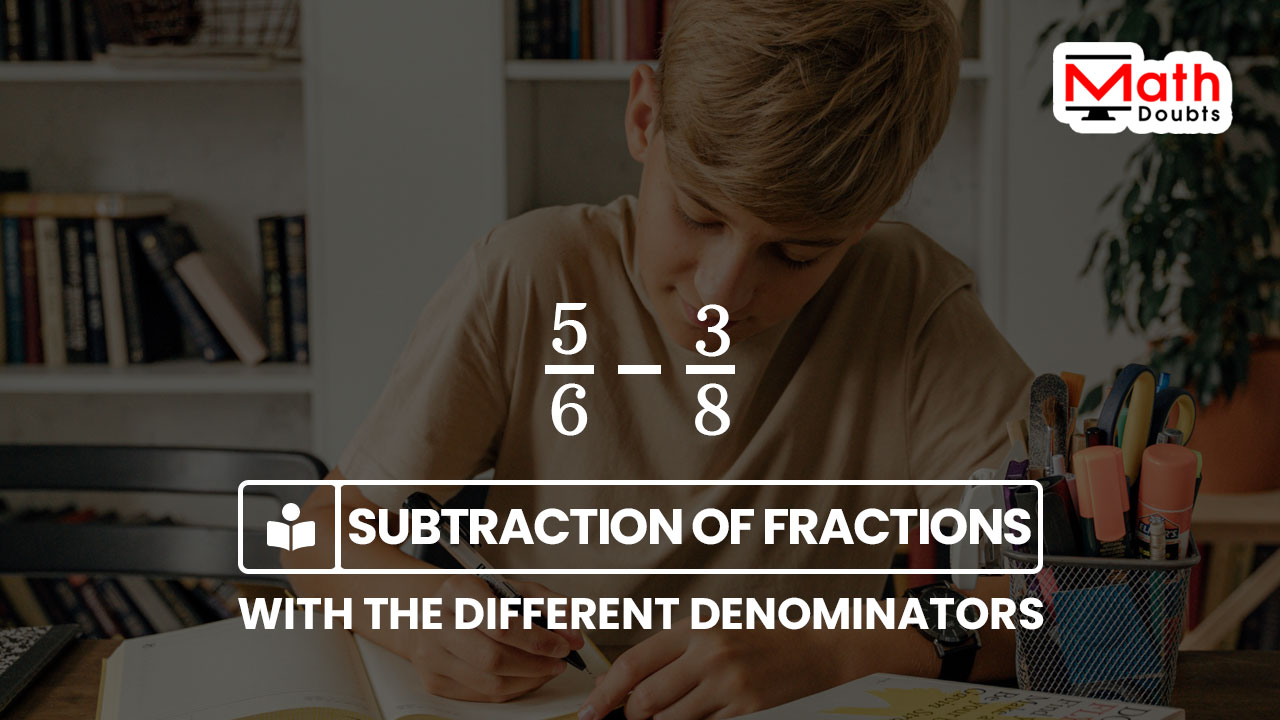# Subtraction of the fractions with the Different denominators

## Definition

A mathematical operation of subtracting a fraction from another fraction with the different denominator is called the subtraction of the unlike fractions.

### Introduction

The minus sign is kept between two fractions to express the subtraction of the fractions in mathematics. In some cases, the denominators of the fractions are different and the mathematical process of finding the difference between such fractions is called the subtraction of the unlike fractions.It is not possible to subtract a fraction from its unlike fraction directly due to different denominators. So, it requires a special procedure to find the difference between them. There are four steps to perform the subtraction of the fractions having the different denominators.

1. Find the LCM of the denominators.
2. Divide the LCM by each denominator to find their quotient.
3. Multiply both numerator and denominator by the respective quotient to convert each fraction in the form of its equivalent fraction.
4. Finally, find the difference between the numerators due to the same denominators.

Now, let’s learn how to use the above four steps to find the difference between the fractions, which have the different denominators.

#### Example

Evaluate $\dfrac{3}{4}-\dfrac{1}{6}$

1. There is a minus sign between the fractions and it indicates the subtraction of the fractions.
2. Look at the denominators, the numbers $4$ and $6$ are different, which means the fractions are unlike fractions.

The above two factors make it clear that the given arithmetic expression expresses the subtraction of two unlike fractions. So, it is time to learn how to find the difference between any two fractions, which have different denominators.

Firstly, let’s find the lowest common multiple of the denominators $4$ and $6$, and their lcm is $12$.

Now, divide the lcm by each denominator of the fractions to find the quotients.

$(1).\,\,\,$ $\dfrac{12}{4} \,=\, 3$

$(2).\,\,\,$ $\dfrac{12}{6} \,=\, 2$

Now, multiply both numerator and denominator of every fraction by the respective quotient to convert them into equivalent fractions.

1. The lcm $12$ is divided by the denominator $4$, and their quotient is $3$. Now, multiply both numerator $3$ and denominator $4$ by the quotient $3$ to convert the fraction $3$ divided by $4$ into its equivalent fraction form.
2. The lcm $12$ is divided by the denominator $6$, and their quotient is $2$. Now, multiply both numerator $1$ and denominator $6$ by the quotient $2$ to convert the fraction $1$ divided by $6$ into its equivalent fraction form.

$\implies$ $\dfrac{3}{4}-\dfrac{1}{6}$ $\,=\,$ $\dfrac{3 \times 3}{3 \times 4}$ $-$ $\dfrac{2 \times 1}{2 \times 6}$

The above arithmetic equation makes it clear that the subtraction of the fractions having different denominators can be evaluated by evaluating the expression on the right hand side of the equation. So, let’s focus on evaluating the arithmetic expression on the right hand side of the equation.

Two numbers are involved in multiplication in both numerator and denominator of every term in the expression. So, let’s find the products of them by multiplication.

$\,=\,\,$ $\dfrac{9}{12}$ $-$ $\dfrac{2}{12}$

According to the subtraction of fractions with same denominator, the difference between like fractions is equal to the difference between their numerators with the same denominator.

$\,=\,\,$ $\dfrac{9-2}{12}$

$\,=\,\,$ $\dfrac{7}{12}$

#### Worksheet

The list of questions on subtraction of fractions having different denominators for practice and solutions to learn how to find the difference between the fractions with the different denominators.

###### Recommended
Latest Math Topics
Jun 26, 2023
Jun 23, 2023

Latest Math Problems
Jul 01, 2023
Jun 25, 2023
###### Math Questions

The math problems with solutions to learn how to solve a problem.

Learn solutions

Practice now

###### Math Videos

The math videos tutorials with visual graphics to learn every concept.

Watch now

###### Subscribe us

Get the latest math updates from the Math Doubts by subscribing us.